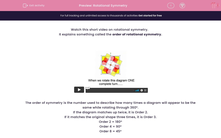# Rotational Symmetry

In this worksheet, students complete diagrams to show rotational symmetry.This content is premium and exclusive to EdPlace subscribers.Key stage:  KS 2

Curriculum topic:   Maths and Numerical Reasoning

Curriculum subtopic:   Symmetry

Popular topics:   Symmetry worksheets

Difficulty level:#### Worksheet Overview

Watch this short video on rotational symmetry.

It explains something called the order of rotational symmetry.

The order of symmetry is the number used to describe how many times a diagram will appear to be the same while rotating through 360°.

If the diagram matches up twice, it is Order 2.

If it matches the original shape three times, it is Order 3.

Order 2 = 180°

Order 4 = 90°

Order 8 = 45°

Example

In the following diagram, what colour must go in square A for the shape to have Rotational Symmetry of Order 2.